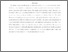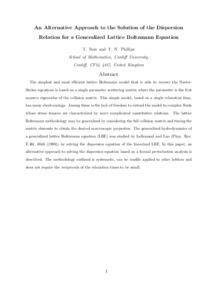# Alternative approach to the solution of the dispersion relation for a generalized lattice Boltzmann equation

and Phillips, Timothy (2008) Alternative approach to the solution of the dispersion relation for a generalized lattice Boltzmann equation. Physical Review E - Statistical, Nonlinear, and Soft Matter Physics, 77 (2):026702. ISSN 1539-3755 (Print), 1550-2376 (Online) (doi:https://doi.org/10.1103/PhysRevE.77.026702)Preview
PDF (Publisher's PDF)
23348 REIS_Alternative_Approach_to_the_Solution_of_the_Dispersion_Relation_2008.pdf - Published Version

## Abstract

The simplest and most efficient lattice Boltzmann model that is able to recover the Navier-Stokes equations is based on a single-parameter scattering matrix, where the parameter is the first nonzero eigenvalue of the collision matrix. This simple model, based on a single relaxation time, has many shortcomings. Among these is the lack of freedom to extend the model to complex fluids whose stress tensors are characterized by more complicated constitutive relations. The lattice Boltzmann methodology may be generalized by considering the full collision matrix and tuning the matrix elements to obtain the desired macroscopic properties. The generalized hydrodynamics of a generalized lattice Boltzmann equation (LBE) was studied by Lallemand and Luo [Phys. Rev. E 61, 6546 (2000)] by solving the dispersion equation of the linearized LBE. In this paper, an alternative approach to solving the dispersion equation based on a formal perturbation analysis is described. The methodology outlined is systematic, can be readily applied to other lattices, and does not require the reciprocals of the relaxation times to be small.

Item Type: Article lattice Boltzmann, multiple relaxation time, dispersion relation Q Science > QA Mathematics Faculty of Liberal Arts & SciencesFaculty of Liberal Arts & Sciences > School of Computing & Mathematical Sciences (CAM) 12 Apr 2019 16:24 None None None None None http://gala.gre.ac.uk/id/eprint/23348View Item